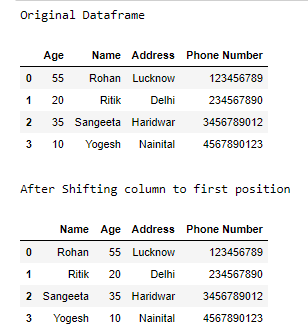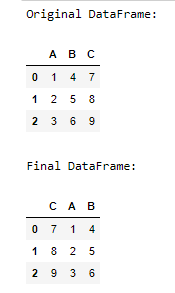# How to Move a Column to First Position in Pandas DataFrame?

• Last Updated : 02 Feb, 2021

Prerequisites: Pandas

The basic idea to move a column in a pandas dataframe is to remove the column from its current place and insert it in the desired position. The pandas library offers many useful functions such as pop() and insert(). We will make use of these two functions to manipulate with our dataframe.

Functions Used:

• Pandas Pop() method is common in most of the data structures but pop() method is a little bit different from the rest. In a stack, pop doesn’t require any parameters, it pops the last element every time. But the pandas pop method can take input of a column from a data frame and pop that directly.

Syntax: DataFrame.pop(item)

Parameters:

• item: Column name to be popped in string

Return type: Popped column in form of Pandas Series

• Pandas insert() method allows the user to insert a column in a dataframe or series(1-D Data frame).

Syntax:

DataFrameName.insert(loc, column, value, allow_duplicates = False)

Parameters:

• loc: loc is an integer which is the location of column where we want to insert new column. This will shift the existing column at that position to the right.
• Column: column is a string which is name of column to be inserted.
• Value: value is simply the value to be inserted. It can be int, string, float or anything or even series / List of values. Providing only one value will set the same value for all rows.
• Allow_duplicates : allow_duplicates is a boolean value which checks if column with same name already exists or not.

Approach:

• Import module
• Remove the column which needs to be shifted to First Position in dataframe using pop() function.
• Insert the column at first position using insert() function.
• Print dataframe.

Let’s understand above approach by below examples:

Example 1:

## Python3

 `import` `pandas as pd`` ` ` ` `# define data``data ``=` `{``'Age'``: [``55``, ``20``, ``35``, ``10``], ``'Name'``: [``'Rohan'``, ``'Ritik'``, ``'Sangeeta'``, ``'Yogesh'``], ``        ``'Address'``: [``'Lucknow'``, ``'Delhi'``, ``'Haridwar'``, ``'Nainital'``], ``        ``'Phone Number'``: [``123456789``, ``234567890``, ``3456789012``, ``4567890123``]}`` ` `# create dataframe``df ``=` `pd.DataFrame(data)`` ` `# print original dataframe``print``(``"Original Dataframe"``)``display(df)`` ` `# shift column 'Name' to first position``first_column ``=` `df.pop(``'Name'``)`` ` `# insert column using insert(position,column_name,``# first_column) function``df.insert(``0``, ``'Name'``, first_column)`` ` `print``()``print``(``"After Shifting column to first position"``)``display(df)`

Output:Example 2:

## Python3

 `import` `pandas as pd`` ` `# define data``data ``=` `{``'A'``: [``1``, ``2``, ``3``], ``'B'``: [``4``, ``5``, ``6``], ``'C'``: [``7``, ``8``, ``9``]}`` ` `# create dataframe``df ``=` `pd.DataFrame(data)`` ` `print``(``"Original DataFrame:"``)``display(df)`` ` `# shift column 'C' to first position``first_column ``=` `df.pop(``'C'``)`` ` `# insert column using insert(position,column_name,first_column) function``df.insert(``0``, ``'C'``, first_column)`` ` `print``()``print``(``"Final DataFrame"``)``display(df)`

Output:My Personal Notes arrow_drop_up